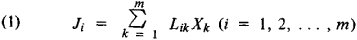Onsager Theorem

Onsager Theorem

one of the fundamental theorems of the thermodynamics of nonequilibrium processes; formulated by L. Onsager in 1931. In thermodynamic systems in which gradients of temperature, component concentration, and chemical potential are present, irreversible processes of thermal conductivity, diffusion, and chemical reactions arise. The processes are characterized by thermal and diffusion fluxes, the rates of chemical reactions, and so on. They are designated by the general term “fluxes” and the symbol Ji; their causes—deviations of the thermodynamic parameters from their equilibrium values—are called thermodynamic forces, Xk. In the case of small thermodynamic forces, the relationship between Ji and Xk can be formulated as a linear equation:where the kinetic coefficients Lik define the contribution of the various thermodynamic forces Xk to the formation of the flux Ji. The relationships (1) are sometimes called the phenomenological equations, and the Lik are called the phenomenological coefficients; the values of Lik are calculated or found empirically. Thermodynamic fluxes and forces may be scalars (as in the case of bulk viscosity), vectors (thermal conductivity, diffusion), or tensors (shear viscosity).

According to the Onsager theorem, if there is no magnetic field and no rotation of the system as a whole, then

(2) Lik = Lki

If an external magnetic field H acts on the system or if the system rotates with an angular velocity ω, then

(3) Lik (H) = Lki(–H) Lki(ω) = Lki(–ω)

The symmetry relationships (2) and (3), sometimes called the Onsager reciprocal relations, establish the relationships among the kinetic coefficients for crossover processes—for example, the relationship between the coefficient of thermal diffusion and the coefficient of the Dufour effect, which is the inverse of thermal diffusion. In the absence of a magnetic field and rotation, these coefficients are equal to one another; specifically, they are equal to the coefficients for chemical crossover reactions.

REFERENCES

de Groot, S. R. Termodinamika neobratimykh protsessov. Moscow, 1956. (Translated from English.)
Denbigh, K. Termodinamika statsionarnykh neobratimykh protsessov. Moscow, 1954. (Translated from English.)
Zubarev, D. N. Neravnovesnaia statisticheskaia termodinamika. Moscow, 1971.

D. N. ZUBAREV

Site: Follow: Share:
Open / Close